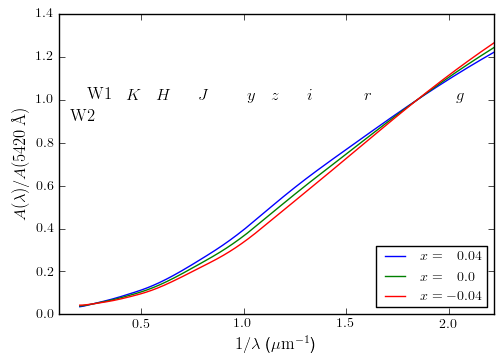We make new measurements of the extinction curve, and have made a new monochromatic extinction curve consistent with these measurements. The curve is normalized to 1 at 5420 Å, and depends on a single parameter, x, which is roughly one-to-one related with R(V). A python implementation of this curve is available here: The following code plots the extinction curve:
import extcurve_s16
ec = extcurve_s16.extcurve(0.) # mean extinction curve
lam = numpy.linspace(5000, 45000., 1000.) # angstroms
plot(lam, ec(lam))
xlabel('$\lambda$ (A)')
ylabel('$A(\lambda)/A(5420 A)$')

Varying R(V) can be obtained by calling extcurve() with different arguments. For example,
ec = extcurve_s16.extcurve(0.04)
roughly corresponds to R(V) = 3.6.